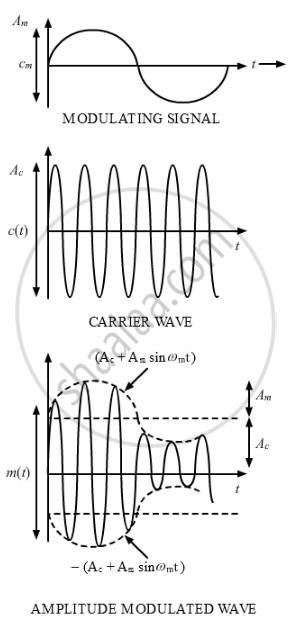Share

Books Shortlist

# Draw a Schematic Sketch Showing How Amplitude Modulated Signal is Obtained by Superposing a Modulating Signal Over a Sinusoidal Carrier Wave. - CBSE (Science) Class 12 - Physics

#### Question

Draw a schematic sketch showing how amplitude modulated signal is obtained by superposing a modulating signal over a sinusoidal carrier wave.

#### Solution

Amplitude modulated signal is obtained by superposing a modulating signal over a sinusoidal carrier wave is shown in the figure given belowIs there an error in this question or solution?

#### Video TutorialsVIEW ALL 

Solution Draw a Schematic Sketch Showing How Amplitude Modulated Signal is Obtained by Superposing a Modulating Signal Over a Sinusoidal Carrier Wave. Concept: Amplitude Modulation.
S